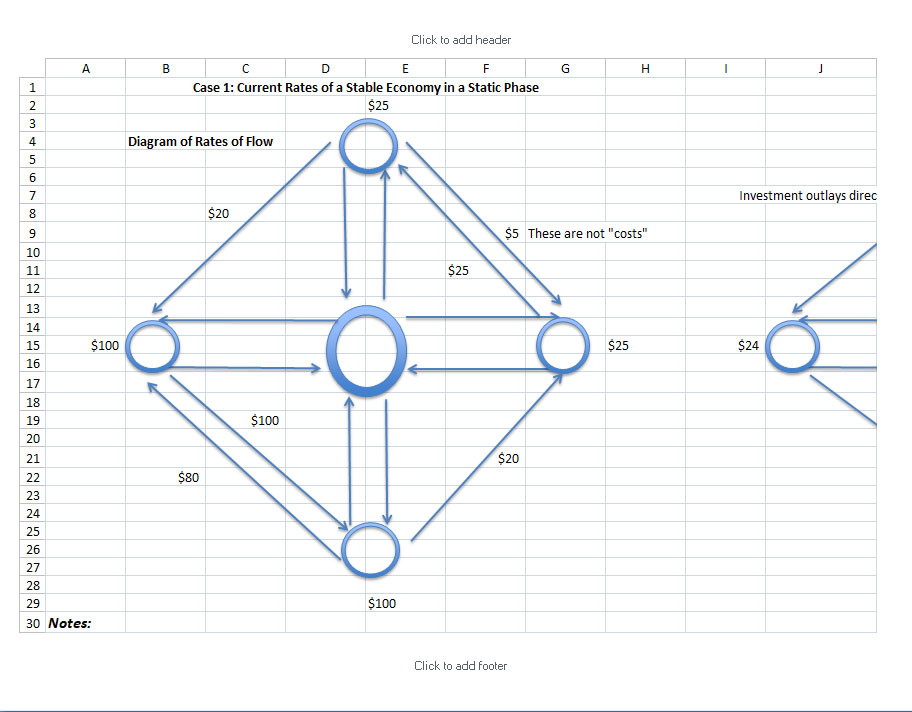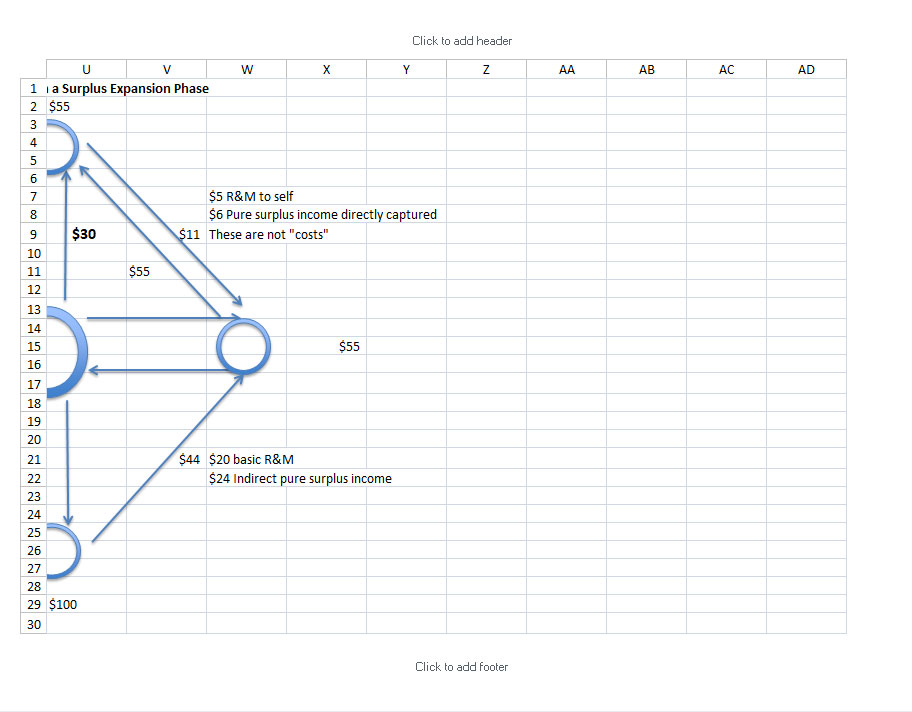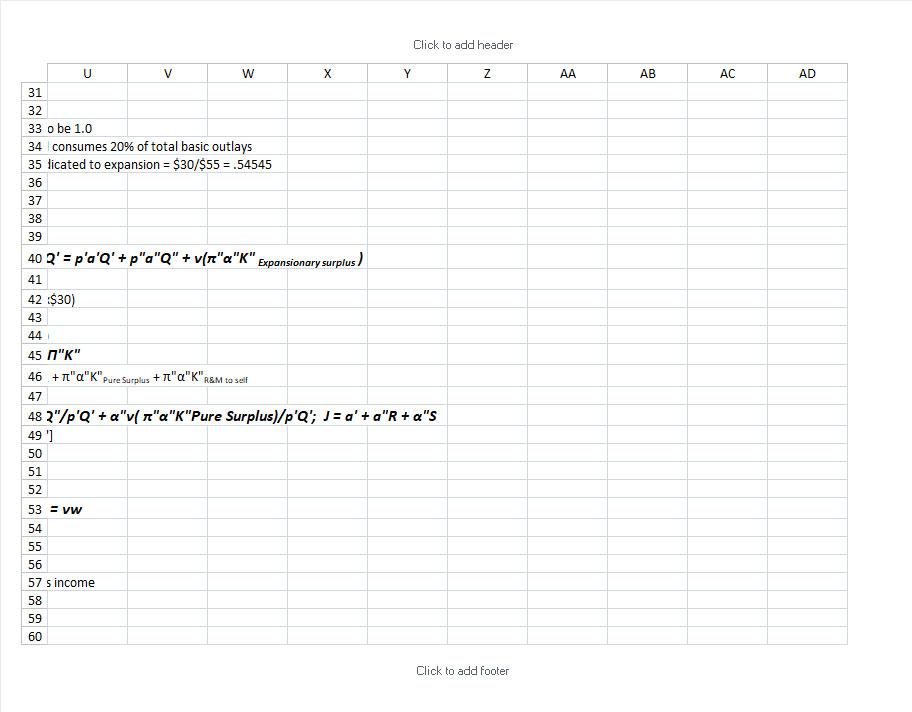### 1.             The Basic Price-Spread Ratio; J = a’ + a”R; The Explanation of Prices

We are doing science.  We are explaining macroeconomic phenomena.  The explanation consists of a coherent system of laws which account for the patterns immanent in the interconnected functionings of the current, purely dynamic, economic process.

Each law is purely relational, and the entire system of laws is coherent.  There are no isolated silos.  No functioning is an island automatic and sufficient unto itself.  The terms of each law define the relations and the relations define the terms.

Thus, masses might be defined as the correlatives implicit in Newton’s law of inverse squares.  Then there would be a pattern of relationships constituted by the verified equation; the pattern of relationships would fix the meaning of the pair of coefficients, m1, m2; and the meaning so determined would be the meaning of the name, mass.  In like manner, heat might be defined implicitly by the first law of thermodynamics (insert) and the electric and magnetic field intensities, E and H, might be regarded as vector quantities defined by Maxwell’s equations of the electromagnetic field.[CWL 3, 80/102-03]

The meaning of the terms representing different functionings is determined by the functional relations of the terms to one another in the collocation of the operators, constants of proportion, and variables in the explanatory equations.  The fundamental terms are functional flows defined by the functional interdependencies in which they stand with one another.

One of the formulae in the system of laws is the Basic Price-Spread Ratio.  This formula does not merely take prices as a given and set them as a priori absolutes without, or regardless of, relations.  Nor does it do what would be even worse: mistake these coincidental or happenstantial prices as the most fundamental terms of systematic significance upon which to construct a comprehensive theory.  That is, the relations of prices to one another do not constitute the primary relativities (or governing integrand) of the system. Rather the formula explains and defines current pricings based upon the prior and more fundamental relativities of the system, the explanatory price-quantity flows.

Frish’s failure to develop a significant theory typifies the failure of economists who search for a dynamic heuristic. As well as a fundamental disorientation of approach there is also a tendency to shift to an inadequate level of abstraction with a premature introduction of boundary conditions in a determinate set of differential and difference equations. [McShane, 1980, 114]

One might be reminded here of a parallel in hydrodynamics: if what is at issue is a general specification of the dynamics of free water waves, a premature introduction of general boundary conditions or worse, specific channel conditions, botches the analytic possibilities….the Robinson-Eatwell analysis is hampered … by their building the economic priora quoad nosof profits, wages, prices, etc., into explanation, when in fact the priora quoad nos are last in analysis: they require explanation. [McShane, 1980,124]

The significance of the basic price-spread ratio P’/p’ cannot be overemphasized.  Few understand the systematics of the basic price-spread ratio. However, it is an explanation removing mystery.

• It expands and contracts systematically in a long-term expansion
• It is connected to the Pure Surplus-Income Ratio, which expands and contracts in a long-term expansion.
• It is intrinsic to an expanding and tapering economy.
• It lays bare the conflict between the criterion of ever increasing earnings and the systematics of production and exchange. Production surges and tapers; and some elements of the sytem expand and contract, while others rise from zero and return to zero.
• It can be used to track, monitor and evaluate the expansion. It can reveal the seeds being sown today of tomorrow’s systematically necessary, corrective depression.
• It explains the process from a special viewpoint.

It is derived as follows:

P’Q’ = p’a’Q’Basic outlays for basic purchases + p”a”Q”Basic R&M outlays circling back for basic purchases

P’Q’/p’Q’ = p’a’Q’/p’Q’ + p”a”Q”/p’Q’

P’/p’ = a’ +a”p”Q”/p’Q’

The form is a ratio equaling the sum of the current basic acceleration factor plus the product of the current surplus acceleration factor times the ratio of current surplus functional “costs” to basic functional “costs.”  To repeat: pricings are determined relationally within a dynamic system of functional flows of factors and money.  They are not simply postulated as a given.  They are explained, and their meaning is determined within the formula which explains them.

Further, the differential of the basic price spread ratio is derived as follows:

P’/p’ = a’ +a”p”Q”/p’Q’

or

J = a’ + a”R

dJ = da’ + a”dR + Rda”

Please study the above derivations from Section 28, The Cycle of the Aggregate Basic Price Spread, [CWL 15, 156-162].

The basic price-spread ratio, i.e. the ratio P’/p’ of selling-price index to cost-price index, expands and contracts systematically during the transition from one level of total output to a higher level of total output.  The expansion and contraction are natural and inevitable, but participants do not understand them as systematically necessary so they do not know how to react to them; they misinterpret them, act according to their survival instinct, and effect the deformation of the normative process.

The  basic price-spread ratio J = a’  + a”R provides the meaning of “prices;” it defines the basic price-spread ratio and its law of change dJ as the process moves through the phases of an expansion; i.e. as a) productive quantities exceed sales quantities (a’or a” or both >1.0), b) sales quantities exceed productive quantities (a’or a”or both < 1.0), and c) as the ratio of surplus “costs” (p”Q”Costs for basic R&Mto basic costs (p’Q’Costs of direct basic factorschanges in the surges of a long-term expansion.  Recall the mathematizable definition of macroeconomic costs in terms of functional flows.

There is a sense in which one may speak of the fraction of basic outlay that moves to basic income as the “costs” of basic production.  It is true that that sense is not at all an accountant’s sense of costs; … But however remote from the accountant’s meaning of the term “costs,” it remains that there is an aggregate and functional sense in which the fraction… is an index of costs.  For the greater the fraction that basic income is of total income (or total outlay), the less the remainder which constitutes the aggregate possibility of profit.  But what limits profit may be termed costs.  Hence we propose ….to speak of (c’O’  = p’a’Q’) and (c”O” = p”a”Q”) as costs of production, having warned the reader that the costs in question are aggregate and functional costs…. [CWL 15, 156-57]

Let us review two simple cases for illustration of a) Gross Domestic Functional Flows, b) the meaning of prices, and c) the meaning of “costs”, and d) the pure surplus income ratio.

Let us jump over to a spreadsheet of images and analyses.  The analysis below the images demonstrates

• The constituents of the flows in the basic circuit P’Q’ =p’ a’Q’ + p”a”Q”
• Gross Domestic Functional Flows
• The Basic Price-Spread Ratio P’/p’ = a’ + a”R
• The Pure Surplus Income Ratio f = vwCase 1 will be a static economy which is running at rates to merely maintain its capacity so as to reproduce itself each period.; a’ = 1, a” =1, p”Q”/p’Q’ = a constant

Basic goods are priced so as to cover the outlays for the material and service components of basic goods plus the outlays required for repair and maintenance to preserve capacity for the next round of production

Case 2 will be comprised of the rates of a surplus-expansion phase in which expansionary investment outlays are not all recaptured directly by investors as a group.  Rather a significant 80% of the investment outlays are directed by the recipients to basic demand for a standard of living.  Thus money which might functionally recycle immediately for another round of expansionary investment passes instead through the basic circuit to increase prices therein.

The reader is strongly encouraged to do one’s own spreadsheet setting up separate cells for each variable on the two formulae P’/p’ = a’ + a”R and f = vw, using absolute references to the variables.  Then, by changing variables the reader can observe how the Basic Price-Spread Ratio and the Pure Surplus Income Ratio change naturally and inevitably as the process advances through the phases of the pure cycle.

The reader is also encouraged to review The  Table of Possibilities of a Given Type below (CWL 15, 114), which indicates in “Row II Neither negative” the phases of an expansion in which there is no contraction or slump and Rows III and V, which indicate how the process might be distorted by negative accelerations.

 dQ”, dQ’ I. Unspecified Surplus Advantage Proportionate Phase Basic Advantage II. Neither negative Surplus Expansion Proportionate Expansion Basic Expansion III. Neither positive Surplus Contraction Proportionate Contraction Basic Contraction IV. Both zero — Mixed Phase — V. One positive, one negative Mixed Phase — Mixed Phase

The basic price-spread-ratio equation ,J = a’ + a”R, explains how the basic price spread P’/p’  expands and contracts during the concrete expansion of the economic process.

F = gm1m2/d2

ΔX E = (-1/c)H’

Δ X H = (+1/c)E’

Δ H = 0

Δ E = 0

The priora quoad nos – first for us – are the things which we notice first because they are related to our sensitive selves, e.g. hot and cold, fast, slow.  The priora quoad se – first among themselves – are the things or terms which are related to each other, e.g. pressure, volume, temperature, space, time, mass, etc.

More fully, the quote is: One might be reminded here of a parallel in hydrodynamics: if what is at issue is a general specification of the dynamics of free water waves, a premature introduction of general boundary conditions or worse, specific channel conditions, botches the analytic possibilities….the Robinson-Eatwell analysis is hampered, not only by an absence of paradigmatic heuristic thinking in a field whose principles involve ends, but also by their building the economic priora quoad nos of profits, wages, prices, etc., into explanation, when in fact the priora quoad nos are last in analysis: they require explanation. McShane, Philip (1980) Lonergan’s Challenge to the University and the Economy, (Washington, D.C.: University Press of America) P. 124

CWL 15, 156-60